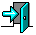# CS3013 Practice Final Exam

1. Consider the `StartUsingProcessTable()` function for the Simple Operating System that we looked at in class. Is there busy waiting? If so, why is it ok here? If not, what kind of waiting is it?

2. Basic memory management:

1. What is the role of the Memory Management Unit (MMU)?
2. If a relocation register had a value of 1200 and a limit register had a value of 1000, give the physical addresses for a logical address of: 20, 850, 1100.

3. Consider a paging system with the page table stored in memory:

1. If the effective memory access time is 200 nanoseconds with paging, how long is the physical (raw) memory access time?
2. If we add associative registers, and we have a 96 percent hit-ratio what is the effective memory access time? Assume access to the associative registers takes 10 nanoseconds.
3. What is the worst-case effective memory access time we could have (using the associative registers from (b))? What is the best effective memory access time we could have (again, using the associative registers from (b))?

4. Fragmentation:

1. What kind of fragmentation do we have with fixed sized partitions? Given N processes each in size M memory partitions how much fragmentation do we have?
2. What kind of fragmentation do we have with variable-sized partitions? Given N processes in memory, how much fragmentation do we have?

5. Consider a system with a 10 bit physical address space divided into 128 frames. The logical address space for each process has 8 pages:

1. How many bits are required for the frame number?
2. How many bits are required for the page number?
3. How many bits are in the offset?
4. How many bits are required for a logical memory address?
5. How big is the page table (in bits) for each process?

6. Consider a system with 32 bits for a memory access. Assume a 2 level paging scheme with 8 bits for the outer page table and 12 bits for the inner page table. How big is each page?

7. Assume pure demand paging. Consider a process with 3 frames of memory and a reference string of `1 2 3 4 1 2 3 4`

1. How many page faults would a FIFO page replacement algorithm have?
2. How many page faults would an LRU page replacement algorithm have?
3. How many page faults would the optimum page replacement algorithm have?
4. Using a working set model with a past reference time of 4, explain why the process is thrashing. What steps might the OS take to help?

8. Explain the principles behind the "top half" of an interrupt handler and the "bottom half" of an interrupt handler.

9. Assume a system using using i-nodes with all file descriptors currently cached in memory. The data to be added is currently in memory, too.

1. What is the minimum number of disk I/O operations (counting each read or write as 1 operation) required to add a block to the beginning of the file?
2. What is the maximum number of disk I/O operations required to add a block to the beginning of the file?

10. Assume in your project 2 (Cloak) assignment you stored a field named `isCloaked` to the ```struct task_struct``` to indicate whether or not a process was cloaked. Place the following pseudo-system calls and statements:

```  p = find_task_by_pid(pid)
if (!p->isCloak)
if (p->isCloak)
uncloak(pid)
proc_register()
sys_uncloak(pid)
cloak(pid)
p->isCloak = 1
Into the following user files: `radar.c` (the module), `cloak.c` (the system calls), `test-cloak.c` (the user level testing of cloaking), `root.c` (the proc filesystem). The calls may be used more than once, as needed.Return to the CS3013 Home Page